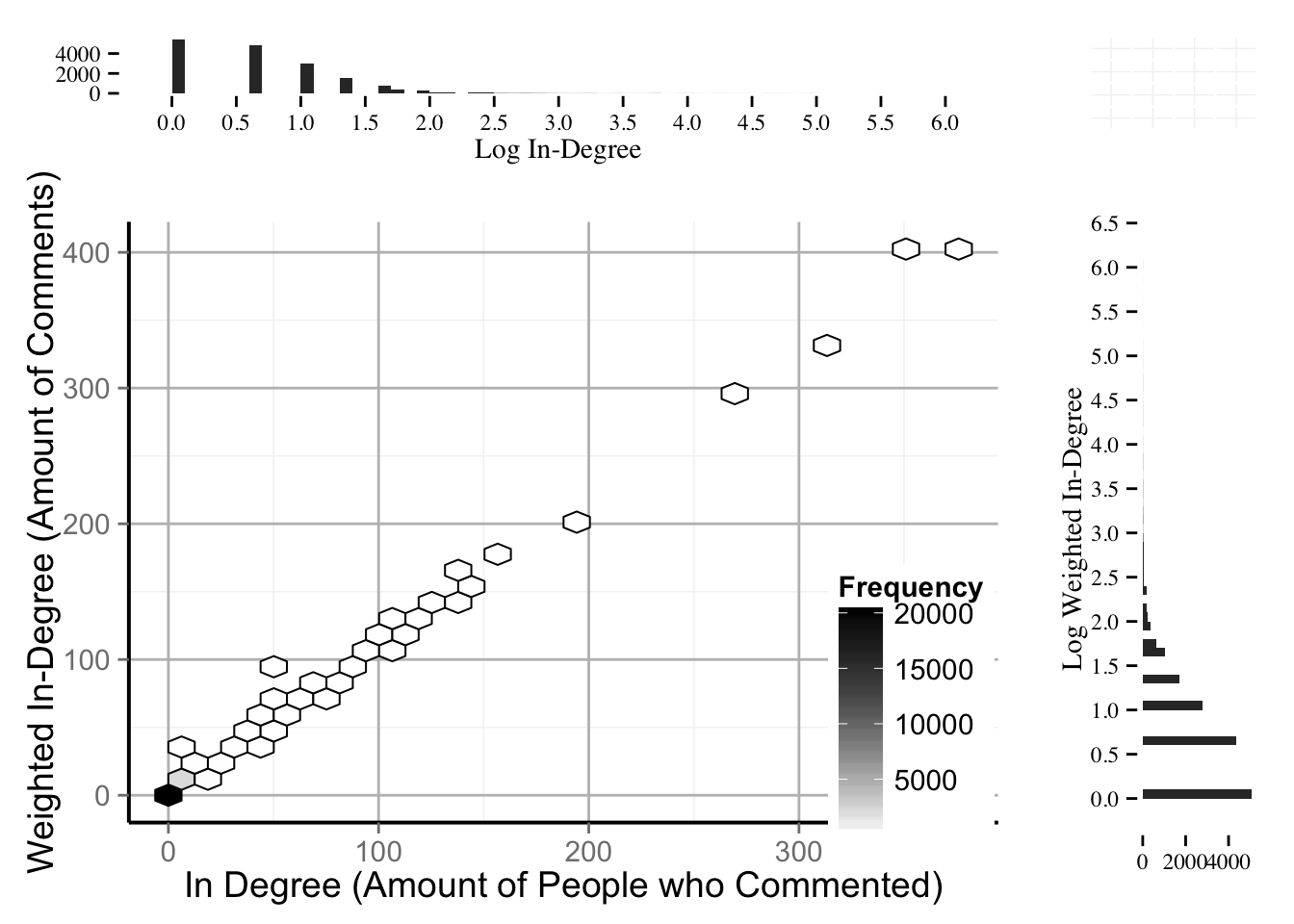#p1 <- ggplot(dat,aes(y=Weighted.In.Degree,x=In.Degree))
#Graph Based on http://stackoverflow.com/questions/11335836/increase-number-of-axis-ticks-in-ggplot2 and http://stackoverflow.com/questions/8545035/scatterplot-with-marginal-histograms-in-ggplot2

#Alpha blending for scatterplot on http://stackoverflow.com/questions/7714677/r-scatterplot-with-too-many-points and http://stackoverflow.com/questions/13167531/ggplot2-multiple-stat-binhex-plots-with-different-color-gradients-in-one-image

library(ggplot2)

#For scatterplot science theme see: http://www.noamross.net/blog/2013/11/20/formatting-plots-for-pubs.html

science_theme = theme(panel.background=element_blank(), panel.grid.major = element_line(size = 0.5, color = "grey"),
axis.line = element_line(size = 0.7, color = "black"), legend.position = c(0.9,
0.2), text = element_text(size = 14))

library(gridExtra)
library(ggthemes)
library(hexbin)
require(scales) #For less ticks

dat <- data[data$type == 'Post',] #Scatter + Side Histograms hist_top <- ggplot(dat, aes(x=log(In.Degree))) + geom_histogram(binwidth=0.1) + theme_tufte() + scale_y_continuous(breaks=pretty_breaks(n=3)) + scale_x_continuous(breaks=pretty_breaks(n=10)) + labs(x="Log In-Degree",y="") empty <- ggplot()+geom_point(aes(1,1), colour="white")+ theme(axis.ticks=element_blank(), panel.background=element_blank(), axis.text.x=element_blank(), axis.text.y=element_blank(), axis.title.x=element_blank(), axis.title.y=element_blank()) #dat <- data[data$type == 'Post' & data$Weighted.In.Degree >= 0,] scatter <- ggplot(dat, aes(x=In.Degree, y=Weighted.In.Degree)) + stat_binhex(colour="black",na.rm=TRUE)+ scale_fill_gradientn(colours=c("white","black"),name = "Frequency",na.value=NA) + #geom_point(alpha = 0.3) + science_theme + guides(legend.position=c(0.2, 0.2)) + labs(x = "In Degree (Amount of People who Commented)", y = "Weighted In-Degree (Amount of Comments)") # scale_x_discrete(breaks=pretty_breaks(n=10)) + #scale_y_discrete(breaks=pretty_breaks(n=3)) hist_right <- ggplot(dat, aes(x=log(Weighted.In.Degree))) + geom_histogram(binwidth=0.1) + coord_flip() + theme_tufte() + scale_y_continuous(breaks=pretty_breaks(n=3)) + scale_x_continuous(breaks=pretty_breaks(n=10)) + labs(x="Log Weighted In-Degree",y="") grid.arrange(hist_top, empty, scatter, hist_right, ncol=2, nrow=2, widths=c(4, 1), heights=c(1, 4))#Calculate Interest Degree #a = data #a$Interest.Degree = data$Weighted.Out.Degree - data$Out.Degree
#write.csv(a, file = "/Users/carlos/Dropbox/Academia/Hawaii/Classes/fall_2014/social_informatics/social_informatics_graphs/flickr_vertex_degree_interest.csv", row.names=FALSE)